Saturday, December 4, 2021
Home > CBSE Class 12 > NCERT Solutions for Class 12 Maths Inverse Trigonometry Exercise 2.1

# NCERT Solutions for Class 12 Maths Inverse Trigonometry Exercise 2.1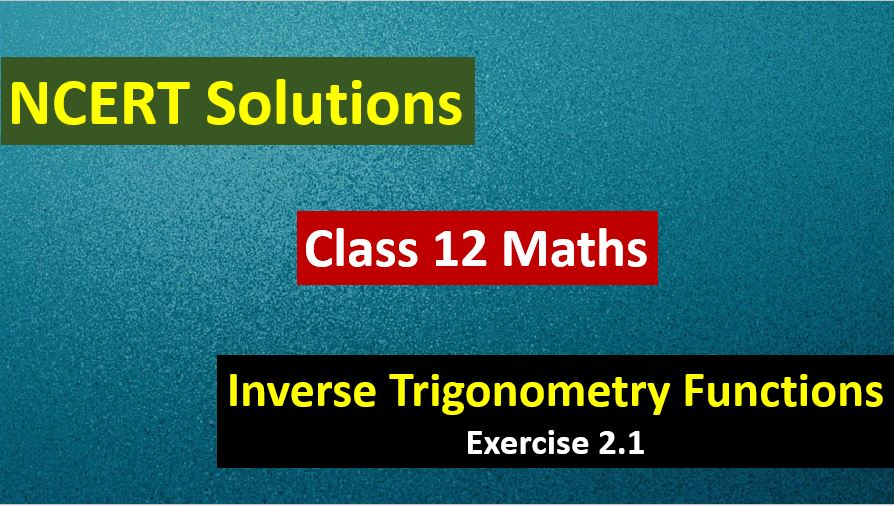# NCERT Solutions for Class 12 Maths Inverse Trigonometry Functions

Hi Students, Welcome to Amans Maths Blogs (AMB). In this post, you will get the NCERT Solutions for Class 12 Maths Inverse Trigonometry Exercise 2.1. This NCERT Solutions can be downloaded in PDF file. The downloading link is given at last.

As we know that all the schools affiliated from CBSE follow the NCERT books for all subjects. You can check the CBSE NCERT Syllabus. Thus, NCERT Solutions helps the students to solve the exercise questions as given in NCERT Books.

CBSE Class 12th is an important school class in your life as you take some serious decision about your career. And out of all subjects, Maths is an important and core subjects. So CBSE NCERT Solutions for Class 12th Maths is major role in your exam preparation as it has detailed chapter wise solutions for all exercise.

NCERT Solutions for Class 12 Maths are not only the solutions of Maths exercise but it builds your foundation of other important subjects. Getting knowledge of depth concept of CBSE Class 12th Maths like Algebra, Calculus, Trigonometry, Coordinate Geometry help you to understand the concept of Physics and Physical Chemistry.

## NCERT Solutions for Class 12 Maths Inverse Trigonometry Functions Exercise 2.1

NCERT Solutions for Class 12 Maths Inverse Trigonometry Exercise 2.1: Ques No 1.Find the principal value of sin-1(-1/2).

NCERT Solutions: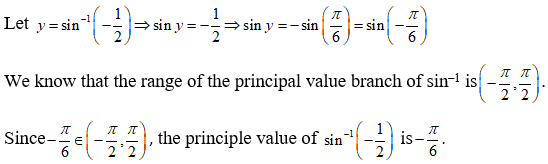NCERT Solutions for Class 12 Maths Inverse Trigonometry Exercise 2.1: Ques No 2.Find the principal value of cos-1(√3/2).

NCERT Solutions: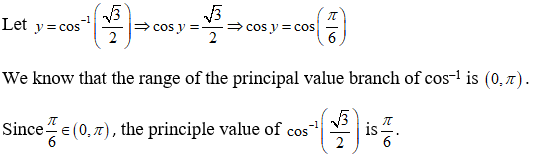NCERT Solutions for Class 12 Maths Inverse Trigonometry Exercise 2.1: Ques No 3.Find the principal value of cosec-1(2).

NCERT Solutions: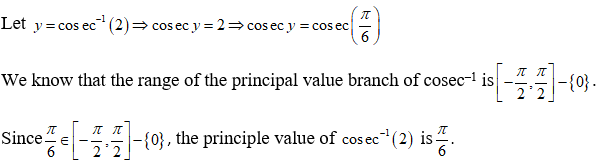NCERT Solutions for Class 12 Maths Inverse Trigonometry Exercise 2.1: Ques No 4.Find the principal value of tan-1(-√3).

NCERT Solutions: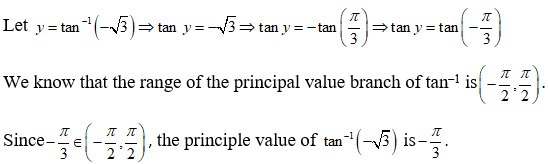NCERT Solutions for Class 12 Maths Inverse Trigonometry Exercise 2.1: Ques No 5.Find the principal value of cos-1(-1/2).

NCERT Solutions:NCERT Solutions for Class 12 Maths Inverse Trigonometry Exercise 2.1: Ques No 6.Find the principal value of tan-1(-1).

NCERT Solutions:NCERT Solutions for Class 12 Maths Inverse Trigonometry Exercise 2.1: Ques No 7.Find the principal value of sec-1(2/√3).

NCERT Solutions:NCERT Solutions for Class 12 Maths Inverse Trigonometry Exercise 2.1: Ques No 8.Find the principal value of cot-1(√3).

NCERT Solutions:NCERT Solutions for Class 12 Maths Inverse Trigonometry Exercise 2.1: Ques No 9.Find the principal value of cos-1(-1/√2).

NCERT Solutions:NCERT Solutions for Class 12 Maths Inverse Trigonometry Exercise 2.1: Ques No 10.Find the principal value of cosec-1(√2).

NCERT Solutions: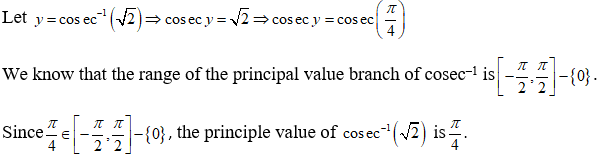NCERT Solutions for Class 12 Maths Inverse Trigonometry Exercise 2.1: Ques No 11.Find the value of tan-1(1) + cos-1(-1/2) + sin-1(-1/2).

NCERT Solutions: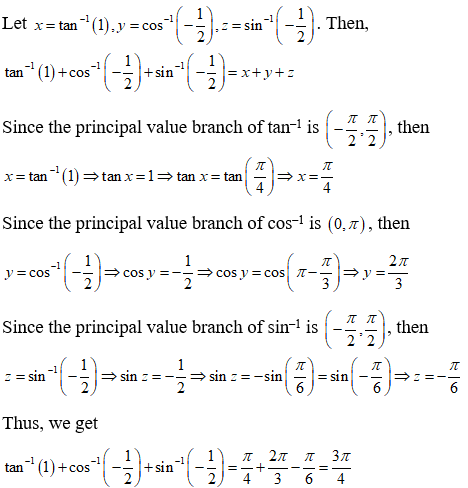NCERT Solutions for Class 12 Maths Inverse Trigonometry Exercise 2.1: Ques No 12.Find the value of cos-1(-1/2) + 2sin-1(1/2).

NCERT Solutions:NCERT Solutions for Class 12 Maths Inverse Trigonometry Exercise 2.1: Ques No 13.If sin-1x = y, thenNCERT Solutions: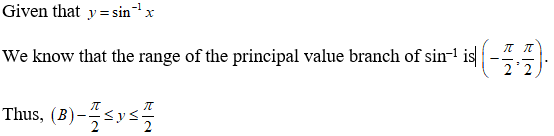NCERT Solutions for Class 12 Maths Inverse Trigonometry Exercise 2.1: Ques No 14.If tan-1(√3) – sec-1(-2) = y, thenNCERT Solutions:AMBiPi
error: Content is protected !!